• ## SFR原理分析 代码

千次阅读 2020-07-07 23:29:20
SFR（spatial frequency response）表示空间频率响应，表示的也是相机的解像能力，在这个层面上，MTF与SFR是一样的意思。 SFR(spatial frequency response ) 主要用于测量随着空间频率的线条增加对单一影像所造成的...
MTF:REF
在表示相机图像解析力时，通常采用MTF50或者MTF50P。 MTF50是当MTF数值下降至最大值的50%时，对应的频率(Cycle Per Pixel)，它是一个广泛应用的锐利度衡量标准。但是它有一个重大的缺陷，就是当影像模组内部的软件对影像作锐利化时，对MTF数值有很大的影响，而其实大部分模组都会对影像作不同程度的锐利化，这就导致了MTF50已经不能够正确的反映锐利度的数值了。
由于前面所述原因，MTF50P被应用在锐利度的评价当中。MTF50P是使影像过度锐化以后再计算MTF数值，其MTF数值的最大值的50%对应的频率值。
SFR（spatial frequency response）表示空间频率响应，表示的也是相机的解像能力，在这个层面上，MTF与SFR是一样的意思。
SFR(spatial frequency response ) 主要用于测量随着空间频率的线条增加对单一影像所造成的影响。简言之SFR就是MTF的另外一种测试方法。这种测试方法在很大程度上精简了测试流程。SFR的计算目标是获得MTF曲线。SFR的计算方法和MTF虽然不同但是在结果上是一致的。 SFR不需要拍摄不同的空间频率下的线对。它只需要一个黑白的斜边（刀口）即可换算出约略相等于所有空间频率 下的MTF。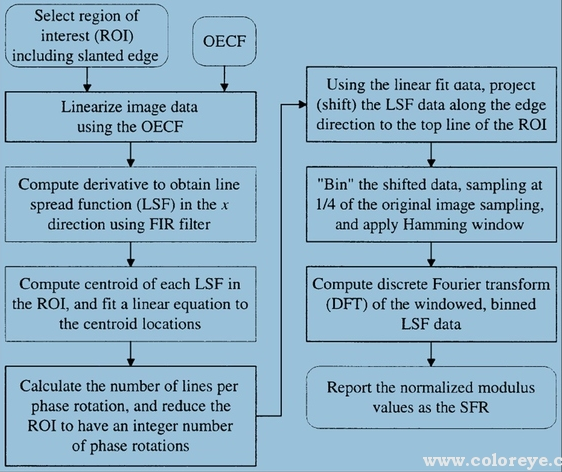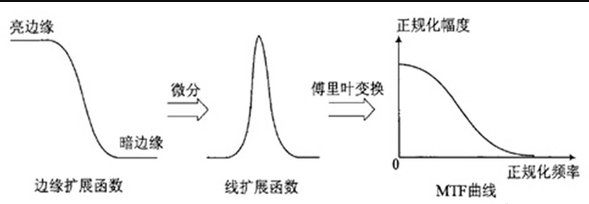SFR是通过这条斜边的图进行超采样的到一条更加细腻的黑白变换的直线（ESF）。然后通过这条直线求导得到直线的变化率（LSF）。然后对将这个变化率进行FFT变换就能得到各个频率下的MTF的值。这里面的ESF，LSF，都是什么呢?
点扩展函数PSF(Point Spread Function)、线扩展函数LSF(Line Spread Function)和边缘扩展函数ESF(Edge Spread Function)是SFR 计算中的几个重要的概念。点扩展函数PSF是点光源成像后的亮度分布函数，如下图所示，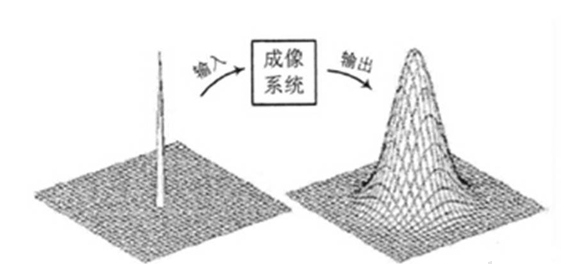用PSF(X,Y)表示。点扩展函数是中心圆对称的，通常以沿x轴的亮度分布PSF(X,Y)作为成像系统的点扩展函数。
当获取点光源像的亮度分布函数PSF(X,Y)后，对其进行二维傅里叶变换即可得MTF (u，v)。因此，从理论上讲，从PSF也是获取MTF的一个方法。但是，在实际的应用中，由于地面点光源强度很弱，此方法一般较少采用。
相对于PSF来说，LSF的能量得到了一定程度的加强。
SFR计​算MTF(通过斜边) 就是通过ESF来得到LSF，然后进行FFT得到MTF各个频率的值的。这几者之间的关系如下图。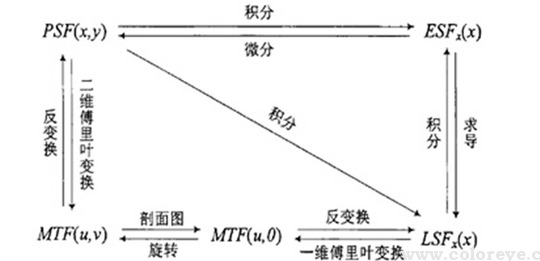算法过程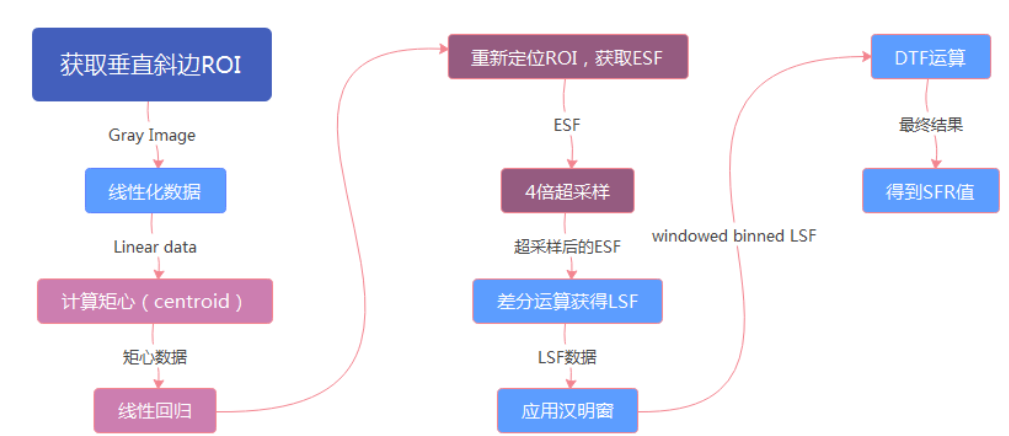0、获取垂直斜边的ROI
1、进行数据的归一化
2、计算图像每一行的像素矩心
3、对每行的矩心使用最小二乘法进行线性拟合，获得一条关于矩心的直线
4、重新定位ROI，获得ESF
5、对获得的ESF进行四倍超采样
6、通过差分运算获得LSF
7、对LSF应用汉明窗
8、进行DFT运算
0 获取垂直边缘的ROI：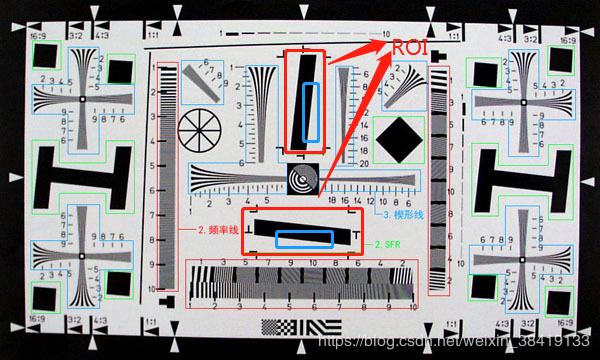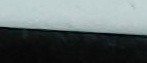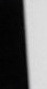这里水平和垂直的Edge只是为了计算图像在水平方向和垂直方向的解析力，与算法本身无关，因为水平的Edge会被进行90°旋转后作为输入，然后计算SFR的值
1、进行数据的归一化
在Sensor获得图像之后，呈现出来的图像由于要符合人眼的感觉，会对图像像素进行伽马变换，使得其变成非线性的像素数据，从而使图像的显示更加符合人眼的感受。所以，当我们要进行sensor的成像解析力分析时，要先将图像处理成没有经过伽马变换前的。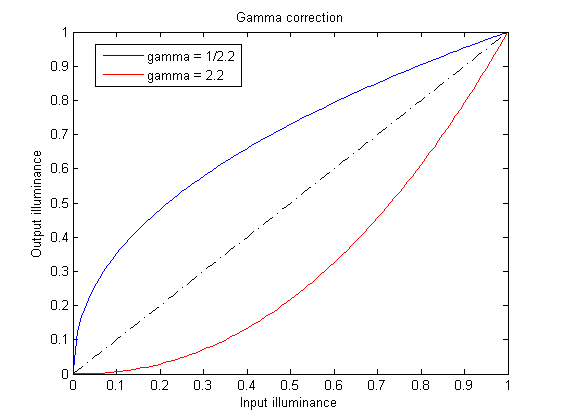一般sensor会对raw图像进行一个2.2的gamma变换，若我们想恢复原始图像时，我们只需要进行一个1/2.2的gamma变换即可。
2、计算图像每一行的像素矩心
这一步的操作其实是为了计算出边缘的位置。具体讲来就是，我们会将图片中的每一行像素都计算具体的矩心位置。
可以看到，其实每一行像素的矩心计算出来的结果，其实就是在黑白分界线的附近。矩心的计算公式如下：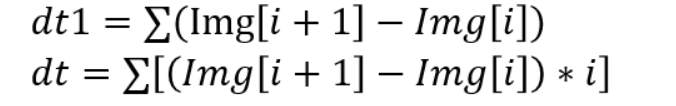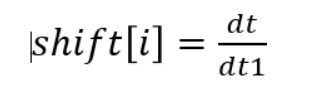shift[i]就是对应的第i行的矩心位置。
/*****************************************************************************/
unsigned short locate_centroids(double *farea, double *temp,
double *shifts,
unsigned short size_x, unsigned short size_y,
double *offset) {
unsigned long i, j;
double dt, dt1, dt2;

/* Compute the first difference on each line. Interpolate to find the
centroid of the first derivatives. */
for (j = 0; j < size_y; j++) {
dt = 0.0;
dt1 = 0.0;
for (i = 0; i < size_x-1; i++) {
dt2 = farea[(j*(long)size_x)+(i+1)] - farea[(j*(long)size_x)+i];
dt += dt2 * (double)i;
dt1 += dt2;
}
shifts[j]=dt/dt1;
}

/* check again to be sure we aren't too close to an edge on the corners.
If the black to white transition is closer than 2 pixels from either
side of the data box, return an error of 5; the calling program will
display an error message (the same one as if there were not a difference
between the left and right sides of the box ) */
if (shifts[size_y-1] < 2  || size_x - shifts[size_y-1] < 2) {
fprintf(stderr,"** WARNING: Edge comes too close to the ROI corners.\n");
return 5;
}
//防止矩心过于靠近图像的边界
if (shifts < 2 || size_x - shifts < 2){
fprintf(stderr,"** WARNING: Edge comes too close to the ROI corners.\n");
return 5;
}

/* Reference rows to the vertical centre of the data box */
j = size_y/2;
dt = shifts[j];
for (i = 0; i < size_y; i++) {
temp[i] = (double)i - (double)j;
shifts[i] -= dt;
}
*offset = dt;
return 0;
}

3、对每行的矩心使用最小二乘法进行线性拟合，获得一条关于矩心的直线
我们知道用的是最小二乘法就可以了。最小二乘法公式如下：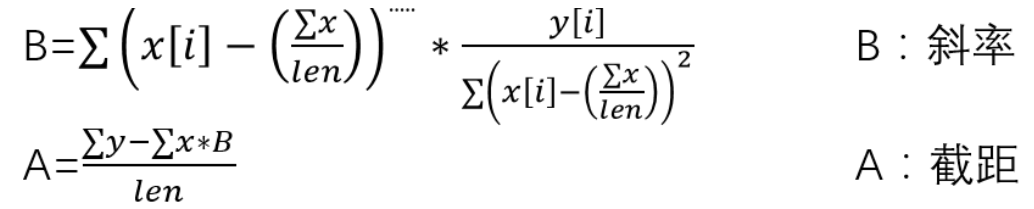unsigned short fit(unsigned long ndata, double *x, double *y, double *b,
double *a, double *R2, double *avar, double *bvar)
{
unsigned long i;
double t,sxoss,syoss,sx=0.0,sy=0.0,st2=0.0;
double ss,sst,sigdat,chi2,siga,sigb;

*b=0.0;
for ( i=0; i < ndata; i++ ) {
sx += x[i];//x的叠加
sy += y[i];//y的叠加
}
//求平均值
ss=(double)ndata;
sxoss=sx/ss;
syoss=sy/ss;
for ( i=0; i < ndata; i++ ) {
t = x[i] - sxoss;  //
st2 += t*t; //方差
*b += t * y[i];
}
*b /= st2;         /* slope  *///斜率
*a =(sy-sx*(*b))/ss; /* intercept *///截距
siga=sqrt((1.0+sx*sx/(ss*st2))/ss);
sigb=sqrt(1.0/st2);
chi2=0.0;
sst=0.0;
for (i=0; i < ndata; i++) {
chi2 += SQR( y[i] - (*a) - (*b) * x[i]);
sst += SQR( y[i] - syoss);
}
sigdat=sqrt(chi2/(ndata-2));
siga *= sigdat;
sigb *= sigdat;
*R2 = 1.0 - chi2/sst;//拟合程度
*avar = siga;
*bvar = sigb;
return 0;
}

4、重新定位ROI，获得ESF
首先，转换坐标轴，将坐标轴转换到计算出来的矩心直线上。如图所示：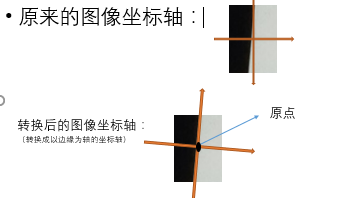/* Start image at new location, so that same row is center */
center_row = *nrows/2;
start_row = center_row - size_y/2;
farea_old = farea;
farea = farea + start_row*size_x;
/* On center row how much shift to get edge centered in row. */
/* offset = 0.;  Original code effectively used this (no centering)*/
if(user_angle)
offset = *off - size_x/2;
else
offset = offset1 + 0.5 + offset2  - size_x/2;

*off = offset;
if(version & ROUND || version & DER3)
offset += 0.125;

if (err != 0)     {   /* Slopes are bad.  But send back enough
data, so a diagnostic image has a chance. */
*pcnt2 = 2*size_x;  /* Ignore derivative peak */
return 4;
}

/* reference the temp and shifts values to the new y centre */
/* Instead of using the values in shifts, synthesize new ones based on
the best fit line. */
col = size_y/2;
for (i=0; i < size_y; i++) {
shifts[i] = (*slope) * (double)(i-col) + offset;
}


5、对获得的ESF进行四倍超采样
然后，我们将每一行中X轴坐标相等的像素值累加起来，然后求均值后得到下面第一行的数组。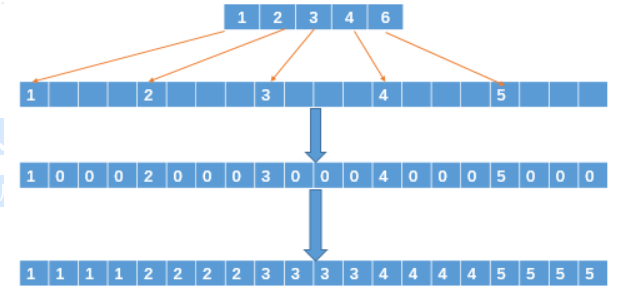这里是将整张图片的像素转换为一个长度为rows的数组，然后进行4倍的扩增。然后其他没有像素的地方，是进行一个向前寻找非0的像素值进行替换，这样就获得了ESF。

//进行超采样，生成长度为size_x*ALPHA(4)的当行图像（ESF），保存在AveEdge中
unsigned short bin_to_regular_xgrid(unsigned short alpha,//alpha->指的是超取样的倍数
double *edgex, double *Signal,
double *AveEdge, long *counts,
unsigned short size_x,
unsigned short size_y)
{
long i, j, k,bin_number;
long bin_len;
int nzeros;

bin_len = size_x * alpha;  //扩大四倍

for (i=0; i<bin_len; i++) {
AveEdge[i] = 0;
counts[i] = 0;
}
for (i=0; i<(size_x*(long)size_y); i++) {
bin_number = (long)floor((double)alpha*edgex[i]);//向下取整
if (bin_number >= 0) {
if (bin_number <= (bin_len - 1) ) {
AveEdge[bin_number] = AveEdge[bin_number] + Signal[i];//把每一行的距离边缘x轴一样远的信号相加
counts[bin_number] = (counts[bin_number])+1;//记录下对应位置有多少个信号相加
}
}
}

nzeros = 0;
for (i=0; i<bin_len; i++) {
j = 0;
k = 1;
//感觉写的有点复杂
if (counts[i] == 0) {
nzeros++; //记录有多少个位置是空的，即没有信号
//K的作用：因为这里的信号为0，找到后面第一个不为零的值，赋给当前这个零
if (i == 0) {
while (!j) { //当j==0时，表示此处的信号为0
if (counts[i+k] != 0) {//第一行的元素
AveEdge[i] = AveEdge[i+k]/((double) counts[i+k]);
j = 1;//充当flag。。。为啥不用布尔类型
}
else k++;//直到找到第一个不为零的数字才会停止
}
} else {
while (!j && ((i-k) >= 0) ) { //j==0&&i-k>=0  j==0说明 counts[i]是0  i-k>0说明  k在i前面，找前面不为零的数值赋给AveEdge[i]
if ( counts[i-k] != 0) {
AveEdge[i] = AveEdge[i-k];   /* Don't divide by counts since it already happened in previous iteration */
j = 1;
}
else k++;
}
if ( (i-k) < 0 ) {//k>i,其实联合上面那段，就是：此处的counts[i]累加次数为零，所以AveEdge[i]也就是0，所以要找到附近一个不为零的值赋给AveEdge[i]
k = 1;
while (!j) {
if (counts[i+k] != 0) {
AveEdge[i] = AveEdge[i+k]/((double) counts[i+k]);
j = 1;
}
else k++;
}
}
}
}
else
AveEdge[i] = (AveEdge[i])/ ((double) counts[i]);//如果此处不为零，直接就求个平均值
}

if (nzeros > 0) {//提示信息
fprintf(stderr, "\nWARNING: %d Zero counts found during projection binning.\n", nzeros);
fprintf(stderr, "The edge angle may be large, or you may need more lines of data.\n\n");
}
return nzeros;
}

6、通过差分运算获得LSF
得到的ESF数组元素进行差分，获得LSF。一下是函数公式：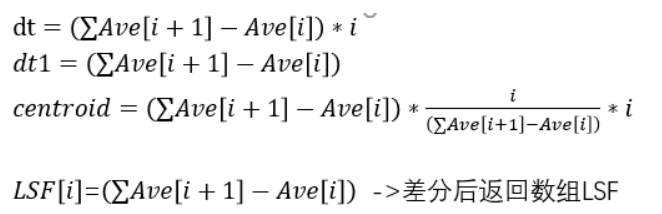void calculate_derivative(unsigned int len, double *AveTmp, double *AveEdge,
double *centroid,int separation)
{
unsigned long i;
double dt, dt1;
dt = 0.0;
dt1 = 0.0;
for (i=0; i< len; i++)
AveTmp[i] = AveEdge[i];

for (i=1; i< len-separation; i++) {
/* Not wasting time with division by 2 since constant factors don't
change SFR computation */
AveEdge[i] = (AveTmp[i+separation]-AveTmp[i-1]);
if (separation == 1)
AveEdge[i] /= 2.0;
dt += AveEdge[i] * (double)i;
dt1 += AveEdge[i];
}
*centroid = dt/dt1;
AveEdge = AveEdge;
if (separation == 1) AveEdge[len-1] = AveEdge[len-2];
}

7、对LSF应用汉明窗
对上面的LSF数组进行汉明窗处理，这一步主要也是看公式，如下：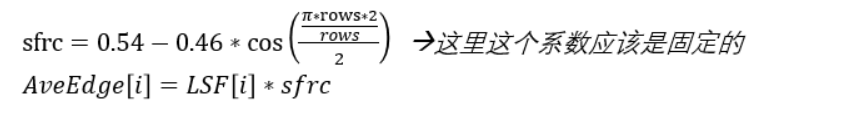void apply_hamming_window(  unsigned short alpha,
unsigned int oldlen,  //size_x*4
unsigned short newxwidth, //size_x
double *AveEdge, long *pcnt2)
{
long i,j,k, begin, end, edge_offset;
double sfrc;

/* Shift the AvgEdge[i] vector to centre the lsf in the transform window */
// 将Avgedge移到中心位置， 两边由于移动造成的空位由0补齐
edge_offset = (*pcnt2) - (oldlen/2);//不能理解为什么一定要反着计算，pcnt2肯定是小于oldlen/2吧。。
if (edge_offset != 0) {                                                   //cer=6
if (edge_offset < 0 ) {  //这里根据分析的话，应该edge_offset只会小于0啊。。     ↓
for (i=oldlen-1; i > -edge_offset-1; i--)            //   [l l l l l l l max l l l l l]
AveEdge[i] = (AveEdge[i+edge_offset]);   //    ↑     ↑        ↑
for (i=0; i < -edge_offset; i++)                     //   left center=3 right
AveEdge[i] = 0.00; /* last operation */    //offset = center-cer=-3
} else {                                               //            cer=6
//              ↓
for (i=0; i < oldlen-edge_offset; i++)               // [0 0 0 l l l l l l l max l l]
AveEdge[i] = (AveEdge[i+edge_offset]);   //  ↑           ↑        ↑
for (i=oldlen-edge_offset; i < oldlen; i++)          //            center=3
AveEdge[i] = 0.00;
}
}
/* Multiply the LSF data by a Hamming window of width NEWXWIDTH*alpha */
//将begin和end两侧的值用0填充,但是感觉没啥用
begin = (oldlen/2)-(newxwidth*alpha/2);//上下看来，begin只会等于0，因为oldlen=bin_len, bin_len=size_x*alpha == newxwidth*alpha
if (begin < 0) begin = 0;
end = (oldlen/2)+(newxwidth*alpha/2);
if (end > oldlen )  end = oldlen;
for (i=0; i< begin; i++)
AveEdge[i] = 0.0;
for (i=end; i< oldlen; i++)
AveEdge[i] = 0.0;

// 给begin和end之间的数据加上汉明窗
// 汉明窗 W(n,α ) = (1 -α ) - α cos(2*PI*n/(N-1)) ，（0≤n≤N-1）
// 一般情况下，α取0.46
// 下面计算方法等于窗发生了平移（故符号发生了变化）， 结果是一样的
for (i=begin,j = -newxwidth*alpha/2; i < end; i++,j++) {
sfrc = 0.54 + 0.46*cos( (M_PI*(double)j)/(newxwidth*alpha/2) );
AveEdge[i] = (AveEdge[i])*sfrc;
}
//将lsfbegin的位置左移到index0的位置
//但在代码中应该是不会起作用的，
if (begin != 0) /* Shift LSF to begin at index 0 (rather than begin) */
for (k=0, i=begin; k<newxwidth*alpha; i++,k++)
AveEdge[k] = AveEdge[i];

}

8、进行DFT运算
DFT公式表达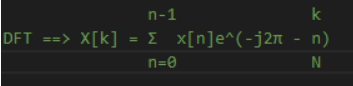最后的到的SFR数组就是空间频域响应的值。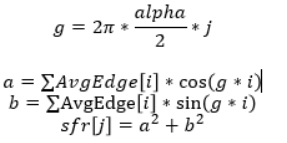unsigned short ftwos(long number, double dx, double *lsf,
long ns, double ds, double *sfr)
{
double a, b, twopi, g;
long i,j;

//                n-1              k
// DFT ==> X[k] = Σ  x[n]e^(-j2π - n)
//                n=0              N

twopi = 2.0 * M_PI;
for (j = 0; j < ns; j++){//ns=1/2*bin_len  前一半
g = twopi * dx * ds * (double)j;
for (i = 0, a = 0, b = 0; i < number; i++) {
a += lsf[i] * cos(g * (double)(i));
b += lsf[i] * sin(g * (double)(i));
}
sfr[j] = sqrt(a * a + b * b);
}
return 0;
}

main
short sfrProc (double **freq, double **sfr,
int *len,
double *farea,
unsigned short size_x, int *nrows,
double *slope, int *numcycles, int *pcnt2, double *off, double *R2,
int version, int iterate, int user_angle)
{
unsigned short i, j, col, err = 0;
long pcnt;
double dt, dt1, sfrc, tmp, tmp2;
double *temp=NULL, *shifts=NULL, *edgex=NULL, *Signal=NULL;
double *AveEdge=NULL, *AveTmp=NULL;
long *counts=NULL;
int nzero;
unsigned short size_y;
unsigned int bin_len;
double avar, bvar, offset1, offset2, offset;
double centroid;
int start_row, center_row;
double *farea_old;
double cyclelimit;
FILE *fp = NULL;

size_y = *nrows;

/* Verify input selection dimensions are EVEN */
if (size_x%2 != 0) {
fprintf(stderr, "ROI width is not even.  Does this really matter???\n");
return 1;
}

/* At least this many cycles required. */
/* For special iterative versions of the code, it can go lower */
if (iterate) cyclelimit = 1.0;
else cyclelimit = 5.0;

/* Allocate memory */
shifts = (double *)malloc(size_y*sizeof(double));
temp = (double *)malloc(size_y*sizeof(double));
edgex = (double *)malloc(size_y*size_x*sizeof(double));
Signal = (double *)malloc(size_y*size_x*sizeof(double));

if( !user_angle ) {
//定位矩心位置
err = locate_centroids(farea, temp, shifts, size_x, size_y, &offset1);
if (err != 0)     { return 2; }

/* Calculate the best fit line to the centroids */
/*计算矩心的拟合曲线*/
err = fit(size_y, temp, shifts, slope, &offset2, R2, &avar, &bvar);
if (err != 0)     { return 3; }

if (version)
MTFPRINT4("\nLinear Fit:  R2 = %.3f SE_intercept = %.2f  SE_angle = %.3f\n",
*R2, avar, atan(bvar)*(double)(180.0/M_PI))
}

/* Check slope is OK, and set size_y to be full multiple of cycles */
//检查刀口斜率，以确保后面超采样的质量
err = check_slope(*slope, &size_y, numcycles, cyclelimit, 1);

/* Start image at new location, so that same row is center */
//调整ROI的行
center_row = *nrows/2;
start_row = center_row - size_y/2;
farea_old = farea;
farea = farea + start_row*size_x;
/* On center row how much shift to get edge centered in row. */
/* offset = 0.;  Original code effectively used this (no centering)*/
if(user_angle)
offset = *off - size_x/2;
else
offset = offset1 + 0.5 + offset2  - size_x/2;

*off = offset;
if(version & ROUND || version & DER3)
offset += 0.125;

if (err != 0)     {   /* Slopes are bad.  But send back enough
data, so a diagnostic image has a chance. */
*pcnt2 = 2*size_x;  /* Ignore derivative peak */
return 4;
}

/* reference the temp and shifts values to the new y centre */
/* Instead of using the values in shifts, synthesize new ones based on
the best fit line. */
// 基于拟合结果更新shifts
col = size_y/2;
for (i=0; i < size_y; i++) {
shifts[i] = (*slope) * (double)(i-col) + offset;
}

/* Don't normalize the data.  It gets normalized during dft process */
//不要对数据进行归一化，数据在DFT处理过程中会被归一化
/* To normalize here, set dt = min and dt1 = max of farea data      */
//如果要在这里初始化，将dt设置为最小值，dt1设置为最大值
dt = 0.0;
dt1 = 1.0;

if (version & ESFFILE)
fp = fopen("esf.txt","w");

/* Calculate a long paired list of x values and signal values */
pcnt = 0;
for (j = 0; j < size_y; j++) {
for (i = 0; i < size_x; i++) {
edgex[pcnt] = (double)i - shifts[j];//计算每个点到刀口的距离
Signal[pcnt] = ((farea[((j*(long)size_x)+i)]) - dt)/(dt1-dt); //归一化每个点的亮度
if ((version & ESFFILE) && edgex[pcnt] < size_x/2 + 3 &&
edgex[pcnt] > size_x/2 - 3)
fprintf(fp, "%f %f\n", edgex[pcnt], Signal[pcnt]);
pcnt++;
}
}

if (version & ESFFILE)
fclose(fp);

/* Allocate more memory */
bin_len = (unsigned int)(ALPHA*size_x);
AveEdge = (double *)malloc(bin_len*sizeof(double));
AveTmp = (double *)malloc(bin_len*sizeof(double));
counts = (long *)malloc(bin_len*sizeof(long));

/* Project ESF data into supersampled bins */
//进行超采样，生成长度为size_x*ALPHA(4)的当行图像（ESF），保存在AveEdge中
nzero = bin_to_regular_xgrid((unsigned short)ALPHA, edgex, Signal,
AveEdge, counts,
size_x, size_y);
free(counts);
free(Signal);
free(edgex);

/* Compute LSF from ESF.  Not yet centered or windowed. */
// 将ESF转换为差分图LSF， 并计算LSF的矩心
if ( (version&DER3) )
calculate_derivative( bin_len, AveTmp, AveEdge, &centroid, 1);
else
calculate_derivative( bin_len, AveTmp, AveEdge, &centroid, 0);

if (iterate == 0) {
/* Copy ESF to output area */
for ( i=0; i<bin_len; i++ )
farea_old[i] = AveTmp[i];
}

/* Find the peak/center of LSF */
//寻找LSF的最大值
locate_max_PSF( bin_len, AveEdge, &pcnt);//这里获得了最大值的中心

free(shifts);
free(temp);

if(version)
MTFPRINT3("Off center distance (1/4 pixel units): Peak %ld  Centroid %.2f\n",
pcnt-bin_len/2, centroid-bin_len/2)

if((version & PEAK) == 0)//忽略差分后的最大值
pcnt = bin_len/2;  /* Ignore derivative peak */
else
MTFPRINT("Shifting peak to center\n")

/* Here the array length is shortened to ww_in_pixels*ALPHA,
and the LSF peak is centered and Hamming windowed. */
//将LSF的最大值移到中心位置，并加上汉明窗
apply_hamming_window((unsigned short)ALPHA, bin_len,
(unsigned short)size_x,
AveEdge, &pcnt);

/* From now on this is the length used. */
*len = bin_len/2;

if (iterate == 0) {
/* Copy LSF_w to output area */
for ( i=0; i<bin_len; i++ )
farea_old[size_x*(int)ALPHA+i] = AveEdge[i];
}

tmp = 1.0;
tmp2 = 1.0/((double)bin_len) ;

/* Now perform the DFT on AveEdge */
/* ftwos ( nx, dx, lsf(x), nf, df, sfr(f) ) */
//ftwos( number, dx, *lsf, ns, ds, *sfr)
(void) ftwos(bin_len, tmp, AveEdge, (long)(*len),
tmp2, AveTmp);

if(*freq==NULL)
(*freq) = (double *)malloc((*len)*sizeof(double));
if(*sfr==NULL)
(*sfr) = (double *)malloc((*len)*sizeof(double));

for (i=0; i<(*len); i++) {
sfrc = AveTmp[i];
(*freq)[i]= ((double)i/(double)size_x);   //每个点对应的频率
(*sfr)[i] = (double) (sfrc/AveTmp);    //归一化sfr
}

/* Free */
free(AveEdge);
free(AveTmp);

*nrows = size_y;
*pcnt2 = pcnt;

return(0);
}

ref https://blog.csdn.net/tanjiaqi2554/article/details/101826860?utm_medium=distribute.pc_relevant.none-task-blog-BlogCommendFromMachineLearnPai2-1.nonecase&depth_1-utm_source=distribute.pc_relevant.none-task-blog-BlogCommendFromMachineLearnPai2-1.nonecase
展开全文• MTF浅谈概论,SFR原理，CTF，各自优缺点 好像很多人很多地方，不管什么样的清晰度测试都通通叫MTF，比如用线对的方式测的对比度也叫MTF，SFR 也叫MTF， 其实如果将MTF作为一个统称概念不是不行，但容易造成很多人混淆...


MTF浅谈概论,SFR原理，CTF，各自优缺点
好像很多人很多地方，不管什么样的清晰度测试都通通叫MTF，比如用线对的方式测的对比度也叫MTF，SFR 也叫MTF， 其实如果将MTF作为一个统称概念不是不行，但容易造成很多人混淆，概念模糊，尤其对于没有光学背景的camera攻城狮来讲，不搞清楚各种方法的概念而统一定性为MTF来进行camera的相关验证和标准的话，恐怕会有不少问题。
参考以下网站介绍，讲的很全面简洁易懂。
http://www.camanalyzer.com/2018/06/14/mtfsfrctfs/

opfun2018, wati, helloword, 598675883, 598675883opoo1705, 598675883VV180330. 598675883VVPT0420, qm20180404, 5109286opfun,zkyby2019, 598675883SK210415, 598675883freever, freeinmainland, mobiles, cameras)
展开全文http
• 奈奎斯特频率Ny = 1/2 * 采样频率 = 1/2 * [1 / (1.12/1000 mm)] = 446.429 (LP/mm) 于是，我们可以根据奈奎斯特频率除以SFR曲线样点宽度，得到各细分频率上的SFR值，如SFR宽度为120样点，则SFR曲线横轴坐标步距为...
本文内容大量援引自如https://wenku.baidu.com/view/c56f2358804d2b160b4ec04a.html等文章和博客的内容，并加入适当个人补充观点，相关内容仅作技术交流讨论，禁止商用，未经授权产生的相关商业纠纷，本人概不负责，若本文观点存在错误，欢迎联系并指正。
1.什么是点扩散函数？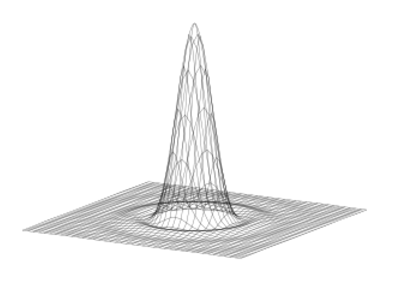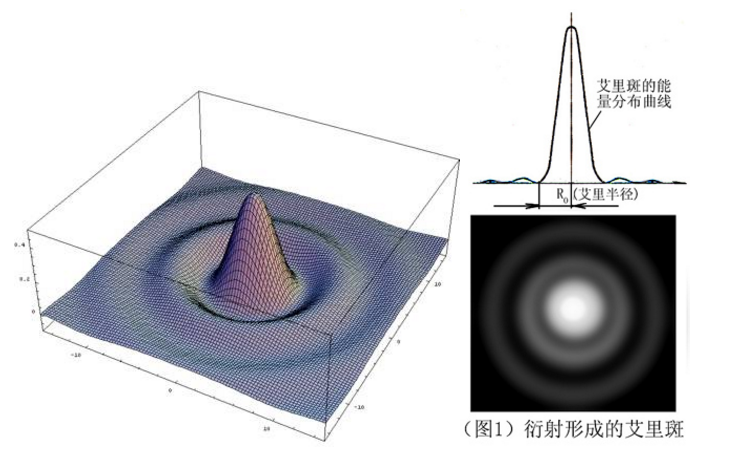上图水平面坐标x,y为像面空间坐标，z轴为像面该点处亮度。
以上摘自博客https://blog.csdn.net/qq254612999/article/details/50509793?locationNum=2&fps=1，
https://blog.csdn.net/glorydream2015/article/details/44966369
由上图我们不难发现点扩散函数的切面图具有如下特性：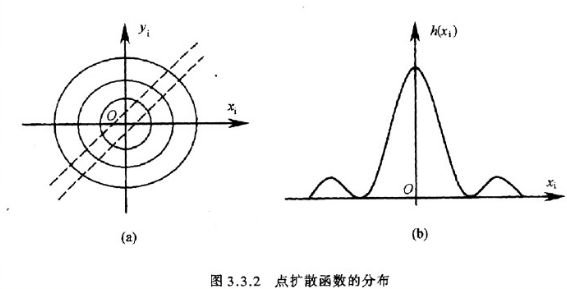在相干光照明时，一个点物在像面上造成的强度分布即为点扩散函数h(xi,yi)。由于像是中心对称的，因此，通过一条过中心的狭缝观察像斑，我们便可以得到如上图b所示的强度分布曲线h(xi),作为沿xi的点扩散函数。

2.什么是线扩散函数？
线扩散函数顾名思义，是由线光源产生，当然你也可以认为是由一排点光源叠加产生。
参见文章http://www.doc88.com/p-6931137520466.html【这篇文章写的很好】，有如下图，小棋认为比较直观的反应了以上内容：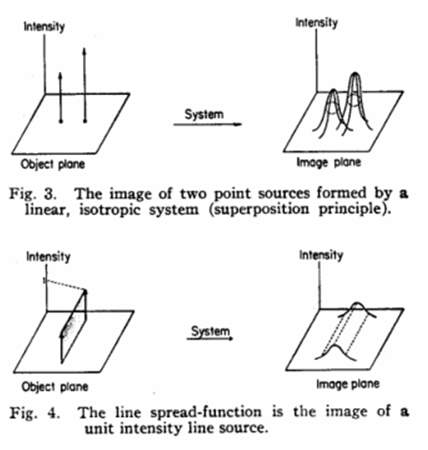如果用亮狭缝或一个亮线通过光学系统成像，取直线像的长度方向为yi，则沿xi方向的光强分布L(xi)就叫做线扩散函数，线扩散函数是由点扩散函数叠加而成。这里小棋不想深入去研究数学模型，读者可以自行阅读小棋分享的几个链接的资料去了解相关内容，以上介绍的成像模型可参考如下图，由此我们发现，其实点扩散函数和线扩散函数从函数外观上是几乎一样的，但注意它们的物理含义是不同的，点扩散函数实际是描述二维的，而线扩散函数侧重于描述一维。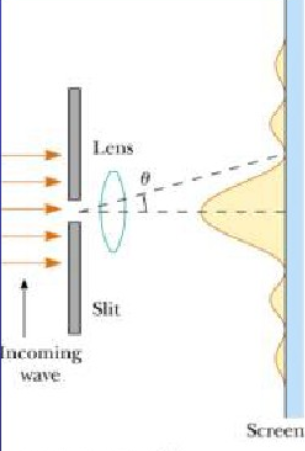3.什么是边沿扩散函数？
有了对线扩散函数理解的基础，请试想一下，假设用一与狭缝方向平行的刀片放置在像平面上。开始时刀片完全挡住狭缝像，逐渐沿xi方向移动刀片，刀片后的探测器接收到的全部光信号与刀片位置关系可用下图的阴影变化表示。光通量随xi的变化称边缘扩散函数E(xi)，与线扩散函数关系为：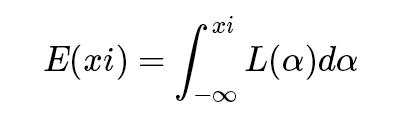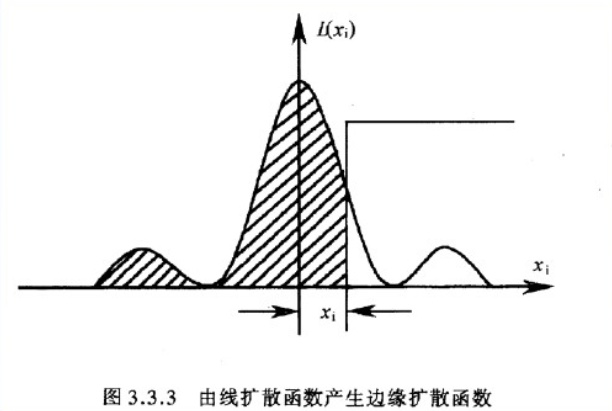对照上图，所谓探测器接收到的总光通量就是图中阴影部分的面积，它是对线扩散函数左半区间内的无穷积分【xi的右侧被刀片挡住，光无法通过】。也即是该处的边缘扩散函数值。因此对线扩散函数积分以后我们有类似如下图的边缘扩散函数：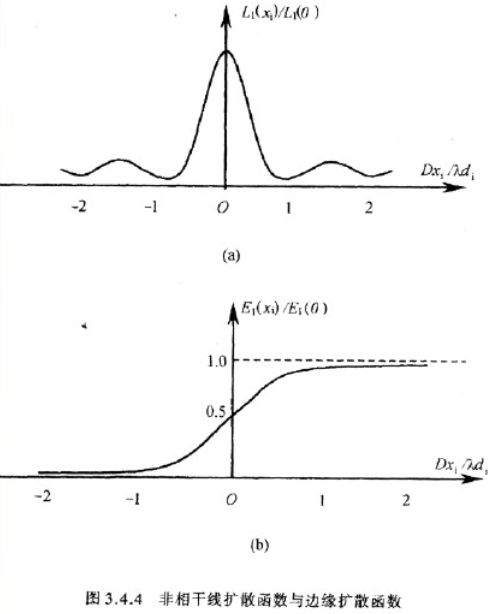接下来，前述文章对线扩散函数做了进一步展开，试想一下，假如我们有两个一强一弱的线光源，这时候发生在像平面上的响应将是这两个线光源的叠加，如下图：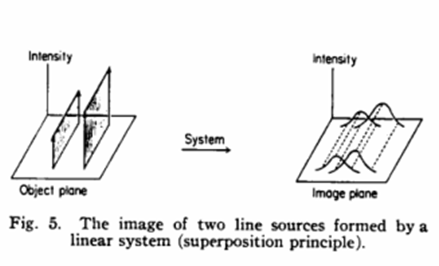但如果我们的实景是如下的样子呢？假设我们的面光源是中心区域亮，两侧区域逐渐变暗。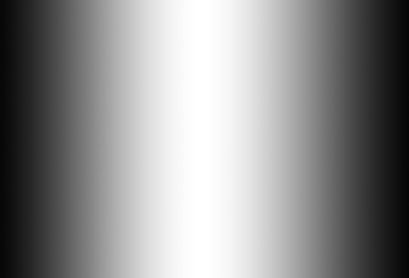这时候我们可以把面中的每一条竖线当成一个假想的线光源，于是我们可以抽象出如下图所示，无限个线光源产生的线扩散函数叠加的像面情形。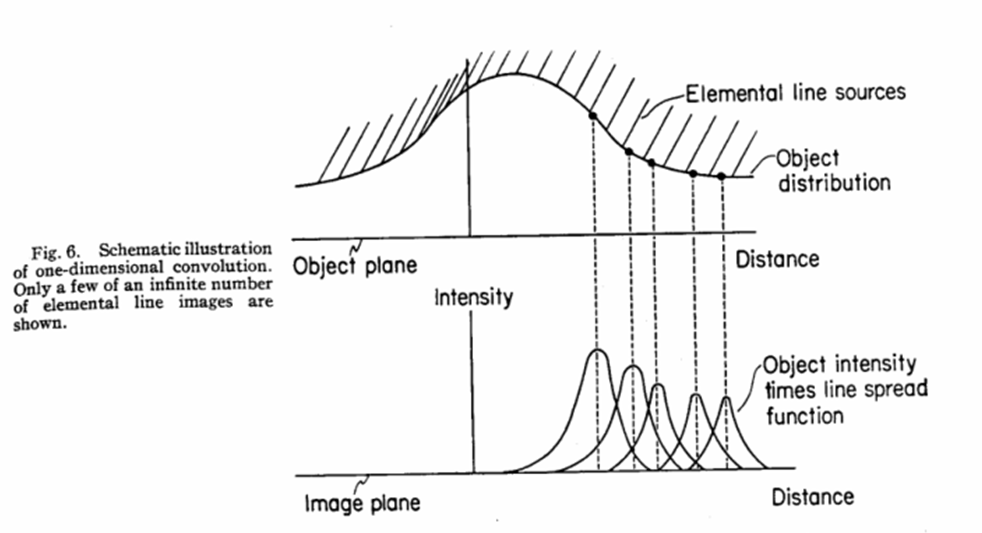接下来，我们回归正题，探讨一下以上内容对于指导斜棱法的意义，由于小棋在上一篇博文中已经提过了ISO12233中是如何通过4倍超采样提升系统亚像素级的采样能力，这里不再赘述，在上一篇中，通过4倍超采样，实际我们对每一行上的像素到斜边矩心距进行了一个加权平均并归档，而归档的过程实际也是一个相位调整的过程，它将原来每一行间互相偏移的相位，又拉回了同一相位上。因此，我们实际上需要探讨的应该是垂直刀口模型。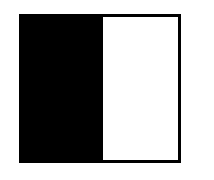同样的抽象方法，我们有如下图的物面和像面响应曲线，我们依旧把物面拆解成无限个线光源的线扩散函数来分析。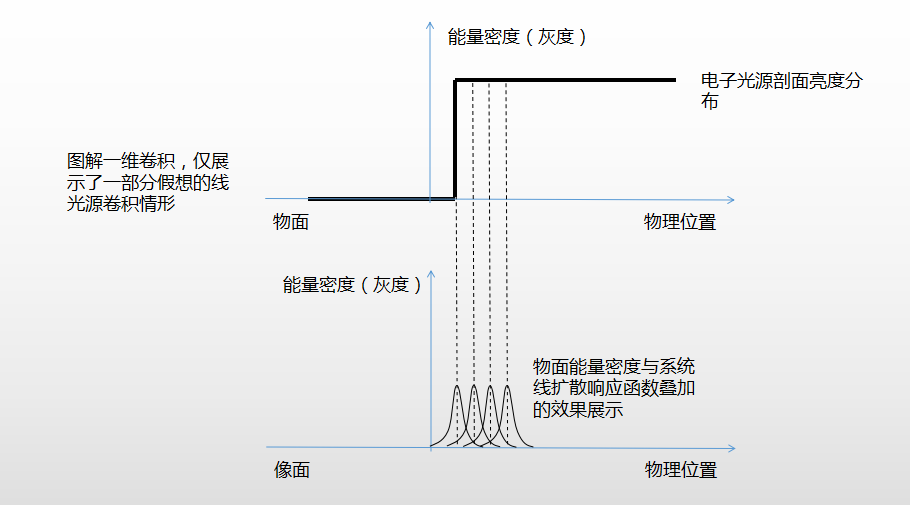上图实际上反应到光学系统中，就是对线扩散函数积分的一个过程，因此反应到4倍超采样的结果上，就是ESF曲线，为什么这么说，下面，小棋进一步图解一下上述过程。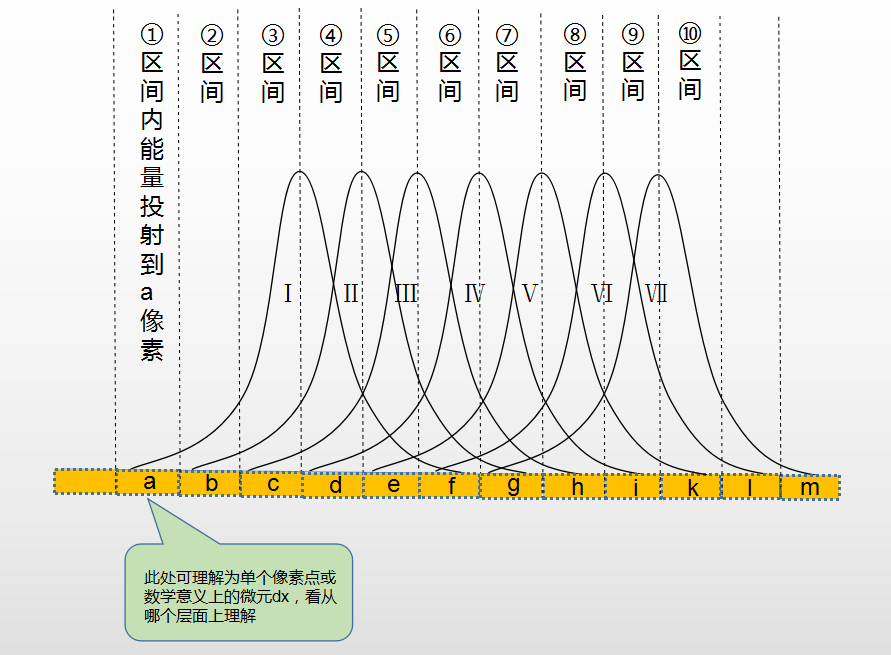如上图，为简化模型，假定线扩散函数间的间距就是一个像素宽度，于是像素点上面包含的所有线扩散函数的面积就是将投影到这个像素点内的光通量的总和，但是它可能来自多个线光源的叠加。
如上图①区间对应的a像素，仅获取到Ⅰ号线光源的部分能量，而②号区间对应的b号像素，则既获取到了Ⅰ号线光源的部分能量，又获取到了Ⅱ号线光源的部分能量，而我们不难发现b像素获取到的Ⅱ号线光源的能量应该等于a像素获取的对应Ⅰ号线光源的能量，原因是我们假定物面是均匀的，即各线光源具有相同的能量密度。
于是我们可以把b像素的两部分能量拼接，也即等价于在②区间左边的所有来自Ⅰ号线光源的能量，显然，后面的情况可以依此类推。
于是投射到各像素上的能量等价于Ⅰ号线扩散函数覆盖区域中，该像素边界处左边所有Ⅰ号线光源能量的积分和，即面积。【两个问题：一、上图最后面有的区间好像缺失了部分能量，那是因为我只画了7条曲线，右边还有无限个，实际情况中也可以再进一步细分出无限个，这里简化了模型。二、像素点到了g以后，就越过了Ⅰ号曲线覆盖范围，显然在分析g像素点时，Ⅰ号曲线的能量已经截止了，这时分析时，应该变更为等价于对应Ⅱ号线扩散函数左边能量的积分和。于是，不难发现，从f开始，后续所有像素均已达到了最大能量密度，即包含整一个线扩散函数的能量密度，f对应Ⅰ号，g对应Ⅱ号，依此类推。】

通过以上分析，请读者再倒回去本文介绍边沿扩散函数时，列举的刀片示例，不难发现，超采样后获得的序列正是边沿扩散函数ESF。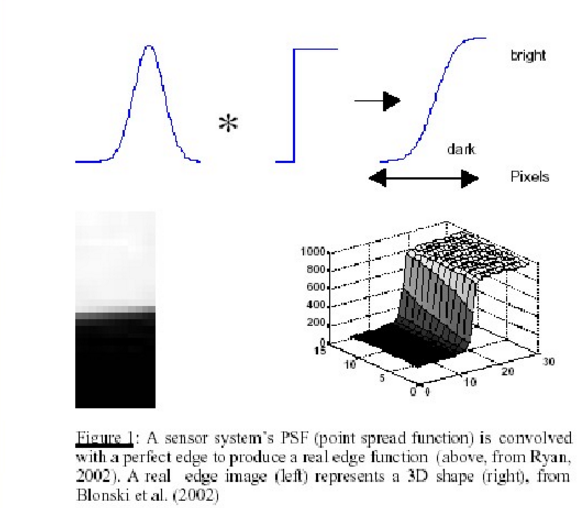此外，上篇博客中提到，ESF卷积一个有限差分滤波器[-1,1]【即微分过程】后，自然得到的也就是线扩散函数LSF。

4.空间频率响应函数SFR的物理意义
我们进一步来分析一下SFR，以及它的物理意义。这里我先给出结论，结论就是对LSF曲线做一维傅里叶变换，就能得到空间频率响应函数e-SFR。
我们知道傅里叶变换可以用来分析时域或空间域的幅频响应特性，显然这里我们分析的是空间域不是时域，我们知道在一幅图像中，有的场景光亮变化很频密，可能在很小的空间内，就发生了频繁的明暗变化，有的场景又变化很缓慢，光处于一个比较柔和的变化中，而我们在此处使用傅里叶变换，就是来分析它针对以上不同空间频率下的一个响应情况，假设它在所有频率上响应都没有衰减，那么它将真实反应实际场景在各个频率下的所有细节。但由于真实的光学系统幅频响应曲线【Fourier Translate{LSF}】逐渐衰减，因此,随着空间频率的升高，最后高频细节将由于混叠而丧失。而通过LSF曲线经傅里叶变换后得到的幅频响应曲线SFR，则正是反应了光学系统对于各种空间频率下的响应能力。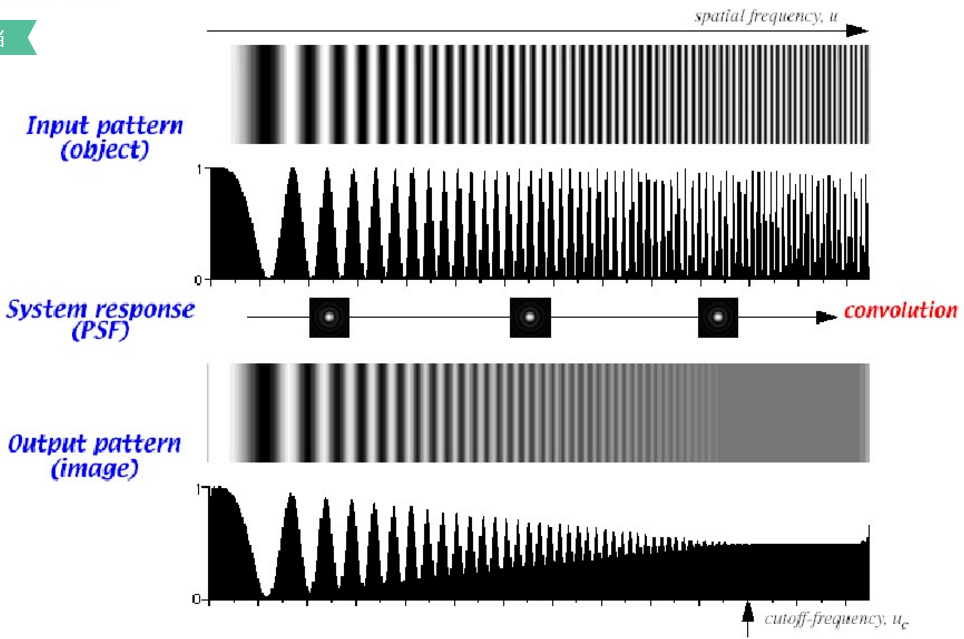如上图，我们控制物面输入的光能量正弦变化【正弦光栅】，且频率越来越高，幅度不变，则得到以上响应输出，以上这种光能量密度正弦变化的标靶，很具有代表性。
我们保持输入为一个同频w的正弦波【对每个单波，波峰一半处为原点】，正弦波sinwt的频谱为两个单位冲激响应【其证明利用了欧拉公式，详见链接文章https://wenku.baidu.com/view/0b7e6f0b783e0912a2162ac3.html?pn=51】，结合之前的分析，该正弦输入会与LSF函数卷积并得到输出图像，而空间域的卷积又等于频域的相乘，正弦波的频谱，仅f0和-f0处有值，其余地方均为0。于是在频域输出上，我们得到的输出频谱将仍是两个冲激响应，只是它的幅度受到了SFR曲线的幅度调制，其逆变换以后，则是一个幅度受LSF影响，产生了一定衰减的同频正弦输出，它的幅度衰减情况与SFR的幅频曲线衰减情况正相关【注意我们这里只讨论了幅频响应，相频变化及相移情况此处未做研究】。因此，我们通过不断调整正弦标靶的空间频率，就可以把SFR曲线上的各个点都测算出来。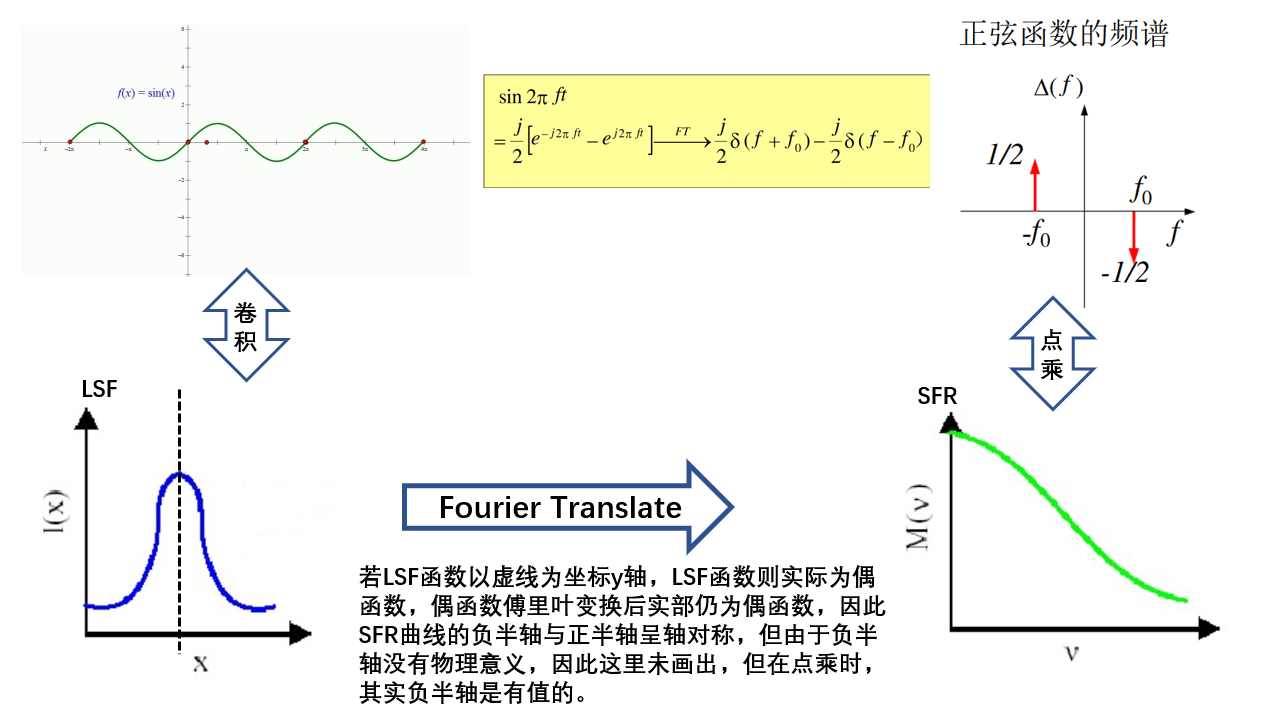但由于上述正弦标靶难于实现，工业上我们不得不妥协，而采用了如下方波输入型【矩形光栅】标靶来近似测算。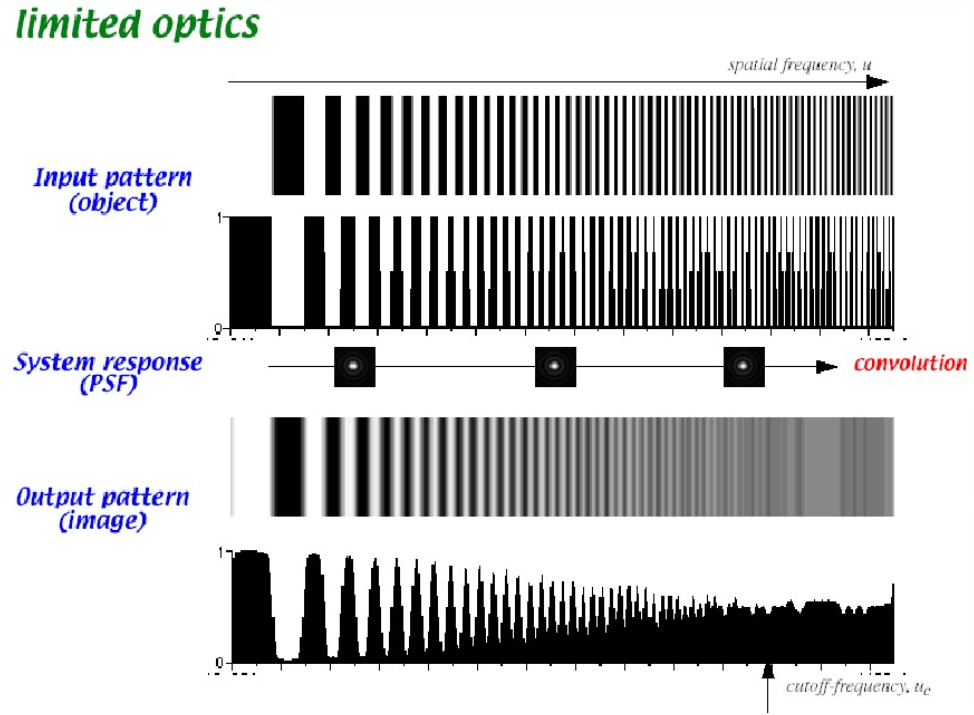最后，结合上一篇博文中计算得到的几条结果曲线，解释一下其横轴的表示方法和单位问题。对于ESF和LSF，横轴坐标可以用水平方向跨越实际像素点的个数表示，又或者是像素点个数的4倍，抑或是用pixel size转换为实际的物理距离。
这里主要讨论一下SFR曲线横轴的几种表现方法，一种是用奈奎斯特频率的倍数，采用这种方式右侧截止位置值为1倍Ny，即1，另一种是采用LP/mm【黑白线对/毫米】的形式【假定pixel size为1..12um,且pixel是正方形的】，有：
奈奎斯特频率Ny = 1/2 * 采样频率 = 1/2 * [1 / (1.12/1000 mm)] = 446.429 (LP/mm)
于是，我们可以根据奈奎斯特频率除以SFR曲线样点宽度，得到各细分频率上的SFR值，如SFR宽度为120样点，则SFR曲线横轴坐标步距为：
步距=采样频率/120= 1000/1.12/120 (LP/mm)= 7.44(LP/mm)
显然此处的曲线最右侧取样点的频率为 119 * 7.44(LP/mm) = 885.36(LP/mm)，但实际上我们只关心截止频率Ny之前的曲线，即小于446.429(LP/mm)之前的数据。
以上，为什么小棋可以这样计算，其实这是离散傅里叶变换的物理意义，建议以离散傅里叶变换DFT或FFT的物理意义为关键词搜索相关内容，或参看以下相关链接，由于控制篇幅，及网上相关时域分析上的介绍资源较多，这里小棋就略去这部分内容了。
http://blog.sina.com.cn/s/blog_90b4c7ff010157gv.html
https://blog.csdn.net/iloveyoumj/article/details/53308142


展开全文• SFR是空间频率响应(Spatial frequency response)的英文缩写，是指一个系统相对于输入的空间频率所输出的振幅响应，对于摄像系统，SFR类似于传统光学系统的MTF（modulation transfer function，调制传递函数），可以...
免责声明：仅供研究讨论，未经确认，相关内容严禁商用，若有错误，欢迎指正。
SFR是空间频率响应(Spatial frequency response)的英文缩写，是指一个系统相对于输入的空间频率所输出的振幅响应，对于摄像系统，SFR类似于传统光学系统的MTF（modulation transfer function，调制传递函数），可以很直观地判定系统的解像能力。

由国际标准化组织ISO（International Standardization Organization）拟定并规范了SFR相关定义及测试方法，其官方网址为：https://www.iso.org/home.html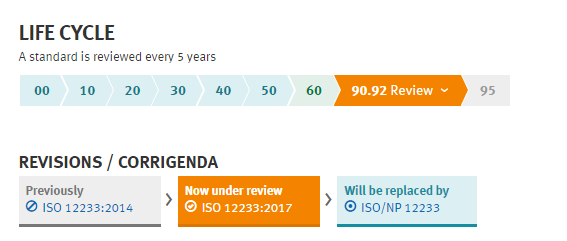SFR的定义及测量方法遵循国际标准ISO12233号文件和图纸，由于2017版文件仍在复核中（网页上述，每5年复核一次标准），这里小编购买的是2014版文件。以下部分内容基于该文件,读者可自行购买该文档。
根据该标准文件，摄像头的解析力和它的SFR由一系列因素决定，其中包含但不限于，镜头的性能，感光器件的可寻址像素数，电路设计（含图像压缩和Gamma校正功能等）。
该文件提出了两种测量SFR的方法：
基于初版修正后产生的斜棱法。基于正弦波方法的SFR新测试方法(星爆图)。

①、测试环境：
测试Chart图的亮度应足以产生一个可接受的摄像头输出信号电平，亮度应均匀分布，且满足任何位置的照度均在Chart图中心照度的±10%以内。光源应被阻挡直射镜头，Chart图周边场景应具有较低反射比，以减小炫光flare.【下图为反射测试环境，也可为透射测试环境，模组厂一般采用背部扩散光源的透射测试模型】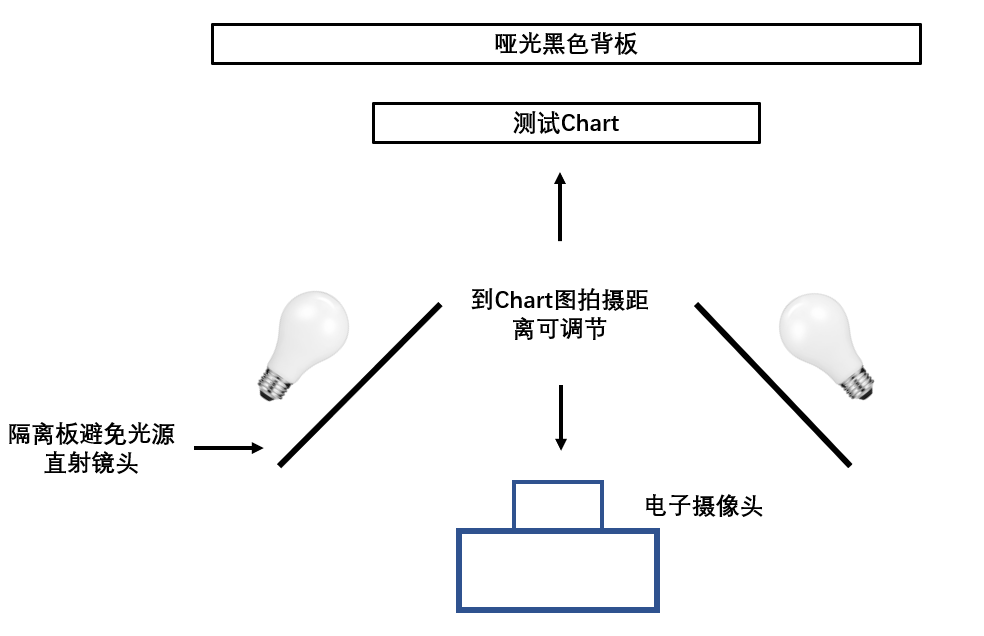从摄像头获取的图像信号可能是非线性的场景亮度值函数。因为SFR是基于线性化的输出信号上定义的测量方法，而这样的非线性信号会影响SFR值，因此，在进行数据分析之前，应先做线性化处理。线性化是通过光电转换函数（OECF）的逆函数来实现的，即通过查找表或应用适当的方程。
②、评判依据
观测者在评判解析力值时应遵守以下规则，建立这些规则的目的是协助我们，在不可避免出现混叠的系统中，获取正确的测量结果。
从低频侧开始，仅当所有相对该空间频率更低的空间频段皆可分辨时，才将该空间频率视为“可分辨”，分辨率的极限定于首次出现混叠，并导致区域产生不可辨的地方。评判某个区域不可分辨的依据是，该处黑白线对极性交换产生混叠，线数模糊，且该处可视线对数少于chart图中该处实际线对数，则我们评判该处“不可辨”。
如下图所示：虚线所指位置处即判定为不可辩识的极限空间频率。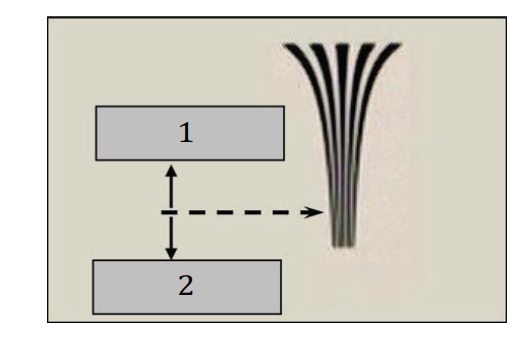展开全文VC++ 图像分析
• 在图像解析力算法—SFR（Spatial Frequency Response）概念理解一文中，我们已经讲解了在阅读SFR源码前必须了解的概念，下面我们来讲解一下，SFR算法的计算具体流程，然后结合源码进行分析， 获取计算公式。...图像处理
• 在图像解析力算法—SFR（Spatial Frequency Response）原理分析(一)中，我们已经分析了SFR的前四个步骤，接下来，我们继续讨论以下的五个步骤 4、重新定位ROI，获得ESF 5、对获得的ESF进行四倍超采样 6、通过差分...图像处理
• SFR是"Spatial Frequency Response"（空间频率响应）的简称，用来表示图形清晰度的算法。本篇文章介绍的的代码实现来自https://github.com/RayXie29/SFR_Calculation，虽然严谨性无法和mitre_sfr算法媲美，但是算法...
• ## 《单片机原理及应用》复习提纲

万次阅读 多人点赞 2015-12-14 10:42:02
《单片机原理及应用》复习提纲 单片机应用系统的典型结构图   单片机应用系统核心硬件技术包括： 1.时序 2.中断 3.地址译码   单片机应用系统核心软件技术包括： 1.寻址...
• ## 【图像处理】SFR算法详解1

万次阅读 多人点赞 2016-03-14 20:44:42
这几篇文章写的是SFR算法，主要根据相关的概念及其对应的标准和源码，来看看SFR究竟是什么？这里先简单介绍下一些基本的概念1、什么是MTFMTF算法是分析镜头解像能力的算法，其全称是Modulation Transfer Function...图像处理
• 空间频率响应SFR测试 一 实验目的 1了解数码相机分辨率测试标准ISO12233以及GB/T 19953-2005数码相机分辨率的测量熟悉测试标板构成掌握其使用方法 2了解数码相机空间频率响应SFR的测试原理理解空间频率响应SFR曲线的...
• 最近这一个月在搞SFR算法--（空间频域响应），终于也算是搞出来了，网上关于SFR计算MTF的资料和博客也是比较少，现在就是总结一下，也算是方便后人，篇幅估计会比较长，会分篇慢慢写。 讲到SFR和MTF，刚入门的小...图像处理
• 相机介绍了锐度，对比度，分辨率，以及MTF曲线的各项意义，并且结合图文的方式，让你能够直观的学习和了解。
• 这个是《【图像处理】SFR算法详解1》的后续，本篇主要讲解SFR算法过程。这里主要参考ISO 12233标准所描述的SFR算法过程，详见《ISO 12233-2000 Camera resolution measurement》中6.3 Saptial frequency response。1...图像处理
• ## 51单片机驱动RC522模块

万次阅读 多人点赞 2019-05-18 21:55:40
想学习使用新的东西时，有必要了解它的工作原理和工作过程，不清楚或者不知道的可以参考相关数据手册和参考文献，在这里为了节省自己的时间，我只对我的51程序做一个小小的笔记~~ 想要驱动RC522模块对IC卡（这里用的...RC522
• 本篇为SFR算法详解系列的第三篇，...这里参考标准中的附录C算法原理及mitre SFR的代码实现，并结合自己需要对其进行优化。1、获取图像ROI这里从拍摄的图像（一般为RGB）中截取ROI区域，并将其转换为Gray。有个问题就图像处理 centroid
• ## 单片机SFR是什么意思？

万次阅读 多人点赞 2020-04-13 13:35:27
SFR全称为：specialfunctionregister（翻译为：特殊功能寄存器） 要想明白什么是sfr，需要先了解什么是寄存器 寄存器是RAM和ROM的统称 就像猫科动物是老虎与狮子的统称一样 ROM或者对于玩电脑的人并不陌生，...
• 本篇为SFR算法详解系列的第四篇，前面三篇为：  《SFR算法详解1》：什么是MTF,MTF50,MTF50P  《SFR算法详解2》：算法过程描述  《SFR算法详解3》：ROI定义，线性化，centroid 本篇主要根据第二篇中的算法...
• 在前面的文章中，我们已经分析了SFR的算法原理与步骤，下面我们直接来分析源码，源码中主要的函数主要分为一下几个： 1、locate_centroids作用：定位每一行像素的矩心位置 unsigned short locate_centroids...图像处理 OpenCV
• 在上一篇图像解析力算法—SFR（Spatial Frequency Response）源码分析(一）中介绍了SFR的几个重要函数，接下来我们看一下主流程和其他函数。 4、sfrProc作用：计算SFR数值的主流程函数 short sfrProc (double **...OpenCV 图像处理 图像清晰度
• sfr特殊功能寄存器 sfr也是一种扩充数据类型，点用一个内存单元，值域为0～255。利用它可以访问51单片机内部的所有特殊功能寄存器。如用sfr P1 = 0x90这一句定P1为P1端口在片内的寄存器，在后面的语句中我们用以用P1......